Try it now!

USD
10

Types of papers:

# The Impact of Wind Power Generation on the Electricity Price in Germany

APA Research Paper

0 / 5

296Click a star to vote!

The Impact of Wind Power Generation on the Electricity Price in Germany (2010-2018)

Name of Student

Institution

The Impact of Wind Power Generation on the Electricity Price in Germany (2010-2018)

Data Description

The data for wind power generation from the year 2010 to 2019 were obtained from European Energy Exchange. The data include the daily prices and production of power in Germany (Ketterer, 2014). This study focused on this time period due to the availability of detailed data. The data presentation of daily wind power (WMh) generated by European Energy Exchange is summarized in Figures 1 to 4. These figures show the distinct outliers in the price series, which may cause “skewness” in the GARCH model.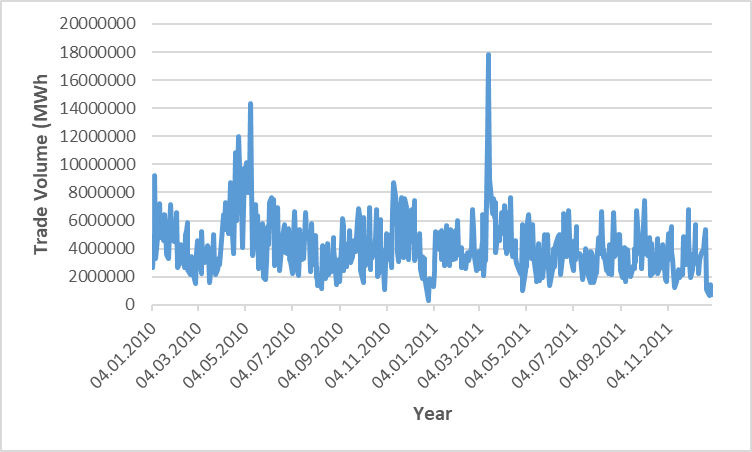Figure 1: The trade volume of wind power in Germany from 2010 to 2011 (European Energy Exchange).

Figure 1 shows various fluctuations in the trade volume of wind power generated during the time period of year 2010 to 2011. The smallest trade volume of 328860MWh was recorded in the year 2010 while the biggest trade volume of 17819299MWh was documented in the year 2011.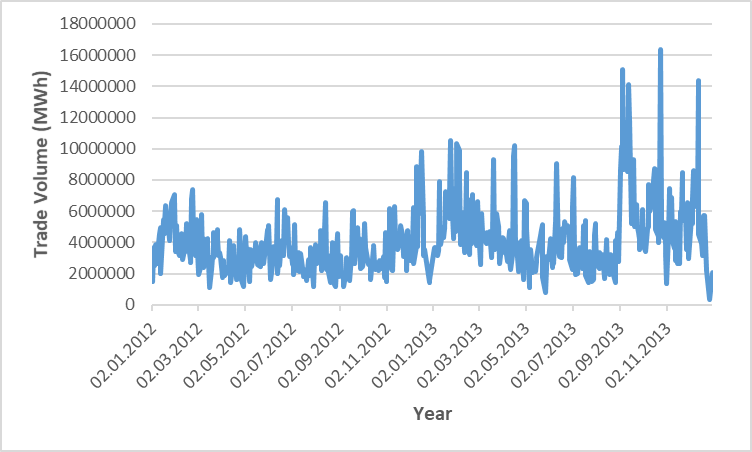Figure 2: The trade volume of wind power in Germany from 2012 to 2013 (European Energy Exchange).

Figure 2 shows the different fluctuations in the trade volume of wind power generated during the time period of year 2012 to 2013. The smallest trade volume of 343623MWh was recorded in the year 2012 while the biggest trade volume of 16372118MWh was documented in the year 2013.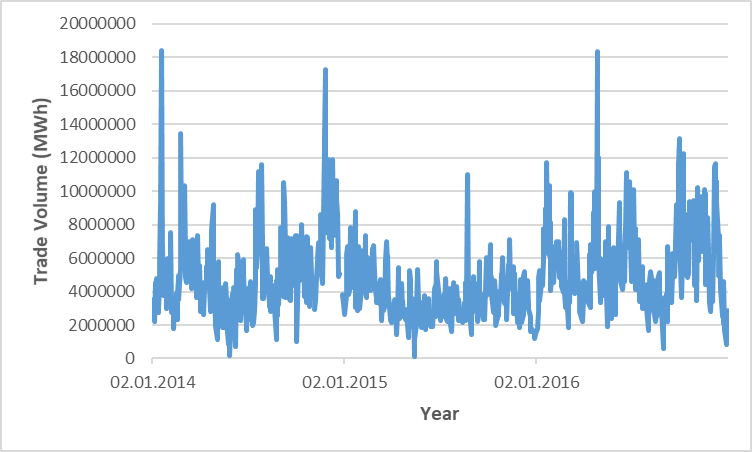Figure 3: The trade volume of wind power in Germany from 2014 to 2016 (European Energy Exchange).

Figure 3 shows the different fluctuations in the trade volume of wind power generated during the time period of year 2014 to 2016. The smallest trade volume of 131520MWh was recorded in May, 2014 while the largest trade volume of 18419754MWh was documented in the year 2016.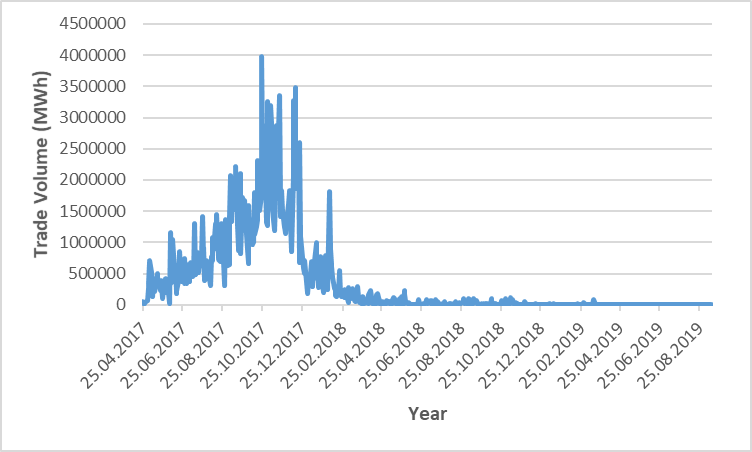Figure 4: The trade volume of wind power in Germany from 2017 to 2019 (European Energy Exchange).

Figure 4 shows the different fluctuations in the trade volume of wind power generated during the time period of year 2017 to 2019. The smallest trade volume of 0MWh was recorded in 2019, while the largest trade volume of 3985726MWh was documented in October, 2017. Moreover, the trend shows that the overall trade volume gradually decreased from the year 2017 to 2019. Figures 5 and 6 show the daily electricity prices (in Euros) in Germany from the year 2010 to 2016. The graphs show the fluctuations in daily electricity prices throughout the year.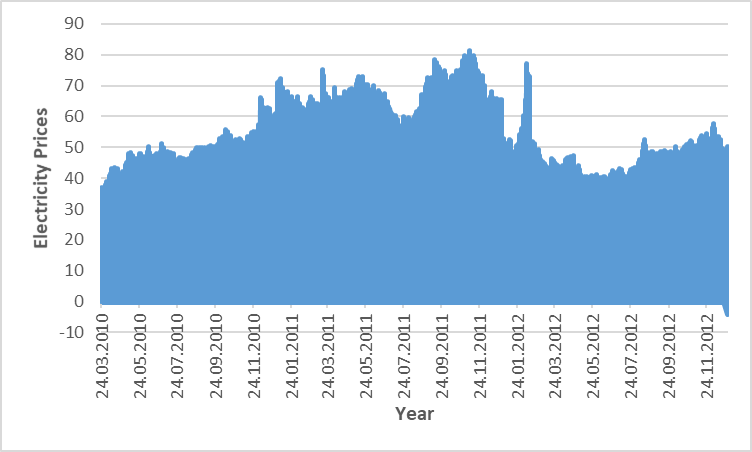Figure 5: The daily electricity prices in Germany from the year 2010 to 2012 (European Energy Exchange).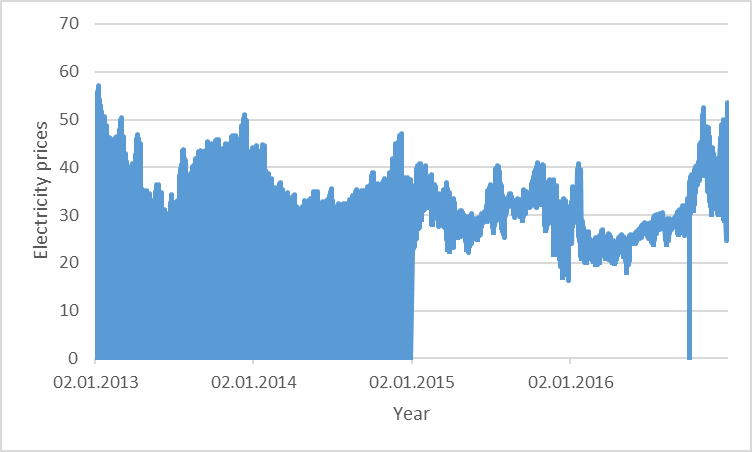Figure 6: The daily electricity prices in Germany from the year 2013 to 2016 (European Energy Exchange).

Reliability and Validity of Data

The data used for this assessment were obtained from secondary sources. Hence, the influence of respondents is insignificant. In addition, the reliability of the data was sustained by removing outliers prior to the application of the GARCH model (Gianfreda, 2010). The spikes in Figures 1 to 4 represent the outliers that cause “kurtosis” in the data distribution. The impact of outliers was reduced without removing any of the observed data. This was carried out by replacing the outlier with a threshold value that is three times more than the mean and standard deviation (Bierbrauer et al., 2007; Gianfreda, 2010). In view of this, the mean and standard deviation of the data set were calculated. The trade volume values that were less than or greater than three standard deviations of the data set were also determined (Ketterer, 2014). A total number of 45 outliers were detected in the data set. These values were replaced with a value equal to three standard deviations from the mean. The new data sets are illustrated in the figures below.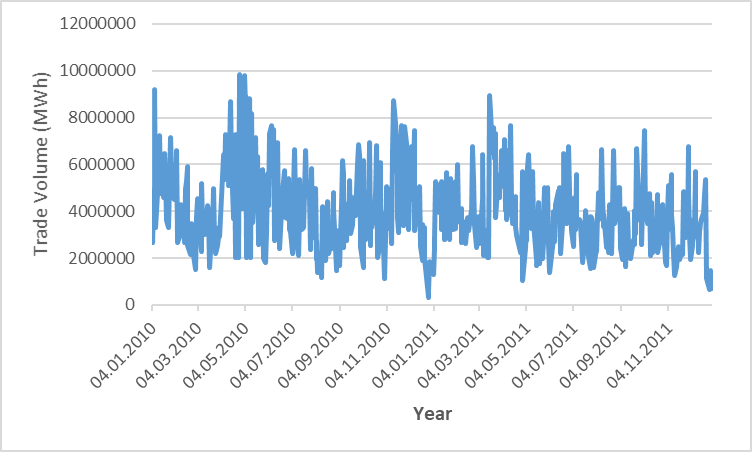Figure 7: The trade volume of wind power in Germany from 2010 to 2011.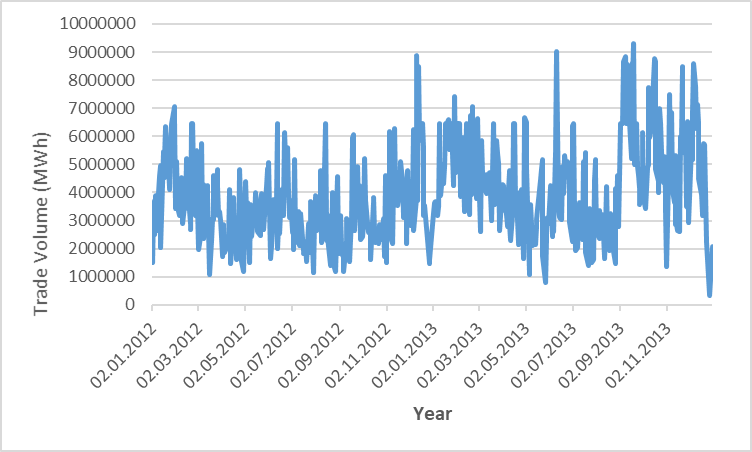Figure 8: The trade volume of wind power in Germany from 2012 to 2013.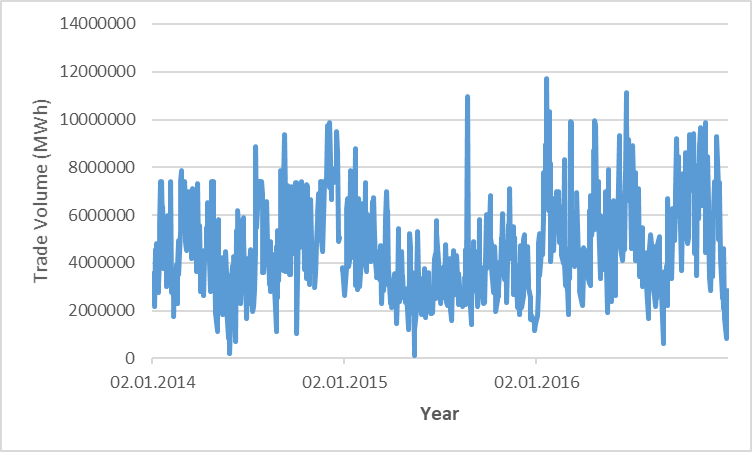Figure 9: The trade volume of wind power in Germany from 2014 to 2016.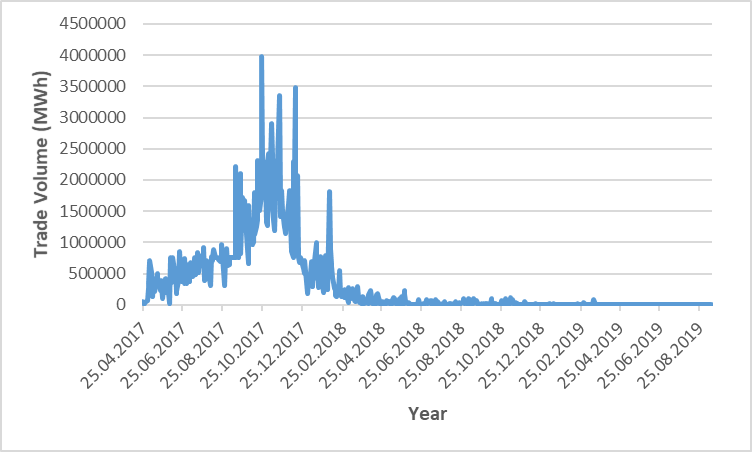Figure 10: The trade volume of wind power in Germany from 2017 to 2019.

The adjusted trade volume values for wind power generated in Germany from 2010 to 2019 are shown Figures 7 to 10. The patterns in each graph look smoother because the spikes have been removed.

Seasonal Variation

Seasonal variation can be described as the effects of varying demands on the price of goods or services. This variation must be addressed prior to subsequent analysis of data (Ketterer, 2014). The mathematical equation for this data is shown below:

𝑝t=𝑦t+𝑠t, where 𝑝t is the price of electricity, 𝑠t is the seasonal part and 𝑦t is the variation component. The seasonal component can be classified as a constant (𝑐), weekday component (𝜉i 𝑑i) and monthly component 𝜈j𝑚j. 𝜉i and 𝜈j are parameters and 𝑑i and 𝑚j are daily and monthly dummy variables. The values of 𝑖 ranges from 1 to 7 while that of 𝑗 ranges from 1 to 12. Using these parameters, the seasonal variation component is shown below:

st=c +i=17εidi+j=112vjmj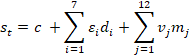The effect of seasonal analysis was reduced by carrying out an Ordinary Least Square (OLS) regression analysis with monthly and weekly dummy variables. In view of this, dummy variables were created for January to December and Monday to Sunday. This test was used to generate the seasonal component of price 𝑝t. The results obtained were used to derive the seasonally adjusted data values.

GARCH Model

The Generalized Autoregressive Conditional Heteroscedastic (GARCH) model was used to investigate the changes in price volatility over a specific period of time. This model was selected for this analysis because it provides reliable information about the short term and long term effects of price volatility (Ketterer, 2014). The GARCH model is suitable for assessing seasonal effects because it relies on autoregressive terms and captures the impact of previous shocks (Pereira & Rodriguez, 2015). The GARCH model can be written as:

yt=μ+i=1liyt-1+j=1mθj  wt-j+ϵt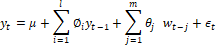ht=ω+i=1qαiϵt-12   +j=1pβjht-j+k=1sγkwt-k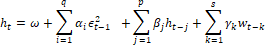In both equations, yt represents the mean equation (in Euros) while ht represents the variance equation (in Euros). In the first equation, 𝜇 is a constant while 𝜙i is the autoregressive term from period i. The natural logarithm for the price of electricity from time period t-1 is denoted as yt-1., while the parameter for wind power production is represented as wt-k. The natural logarithm was selected based on its effect on elasticity. The weekday effects were investigated by using 7 different lags in the equation.

On the other hand, the conditional variance equation 𝜔 denotes the value for the long-term variance. The coefficients 𝛼 and 𝛽 show the effect of current and past shocks on the volatility of prices. The 𝛾 coefficient is the parameter for the wind power generated. The error term 𝜖 is calculated as htzt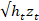. Each electricity market is characterized by a sudden increase in prices, which later change to the mean value due to the effect of the forces of demand and supply. The stability of Germany’s energy market was measured using the conditional variance equation. If the sum of 𝛼i and 𝛽j is less than 1 and the result of multiplication of both parameters is more than 0, it can be concluded that the model is “mean reverting”. This outcome also indicates that the impacts of past and current shocks have a temporary effect on the conditional variance ht. In addition, the role and effects of wind power on the volatility of prices was investigated by carrying out a time series analysis (Ketterer, 2014). The level of significance for this test is 10% (i.e. 90% confidence interval).

Econometric Issues Encountered During the Assessment

The GARCH model was run on a computer using econometric software known as SPSS. However, some of the limitations of this application include the poor quality of default graphics, the absence of information about the effect of confidence intervals and size as well as the unavailability of algorithm documentation. One of the econometric issues encountered while using this software was the non-positive definite warning. However, this notification was considered as insignificant because it occurred only thrice in the data set.

References

Bierbrauer, M., Menn, C., Rachev, S. T., & Trück, S. (2007). Spot and derivative pricing in the EEX power market. Journal of Banking and Finance, 31 (11), 3462-3485.

Gianfreda, A. (2010). Volatility and volume effects in European electricity spot markets. Economic Notes, 39 (1‐2), 47-63.

Ketterer, J. C. (2014). The impact of wind power generation on the electricity price in Germany. Energy Economics, 44, 270-280.

## Related samples

Numerous previously developed lands are currently not in use across the globe. In the US, there are approximately 450,000 brownfields that had either… .

Research Report

0 / 5

306

About 1,253 tornadoes sweep the USA each year (ncdc.noaa.gov, 2016) causing devastation, destruction, chaos, damage and even death. According to the… .

Article Review

0 / 5

1487

Information technology has evolved into one of the critical aspects of the modern organizational structure. Today, enterprises use different systems… .

Engineering Report

5 / 5

1587

USD
10